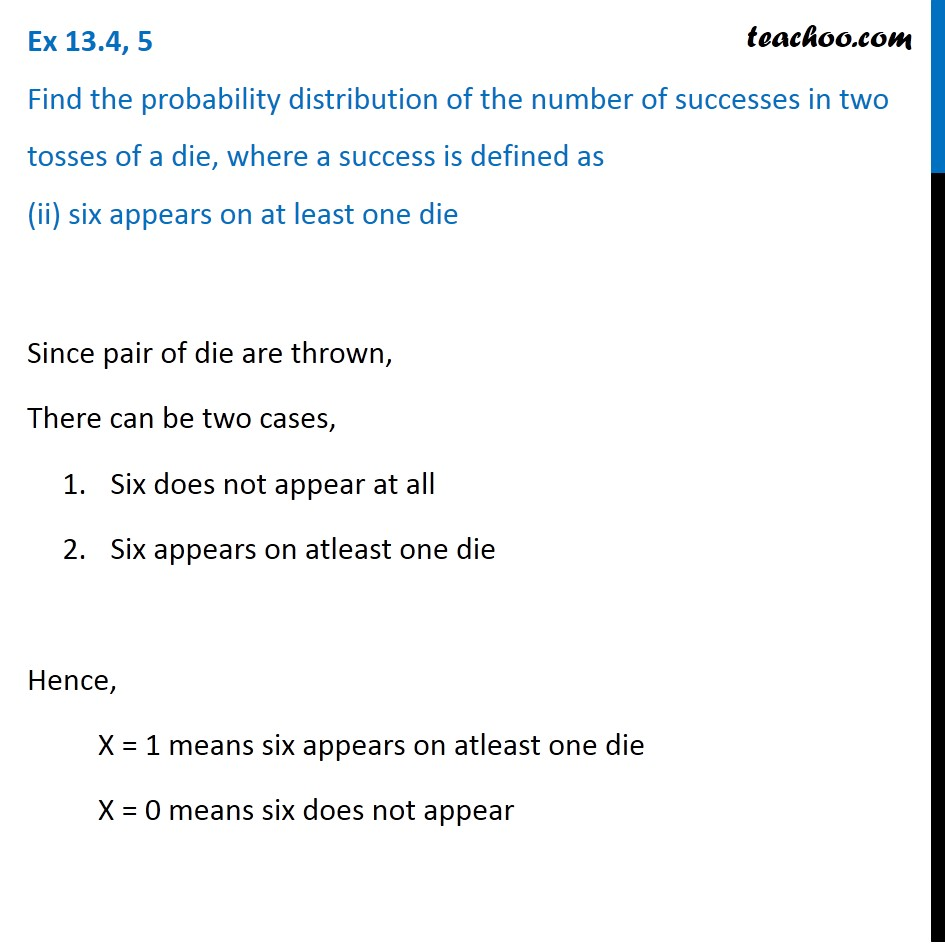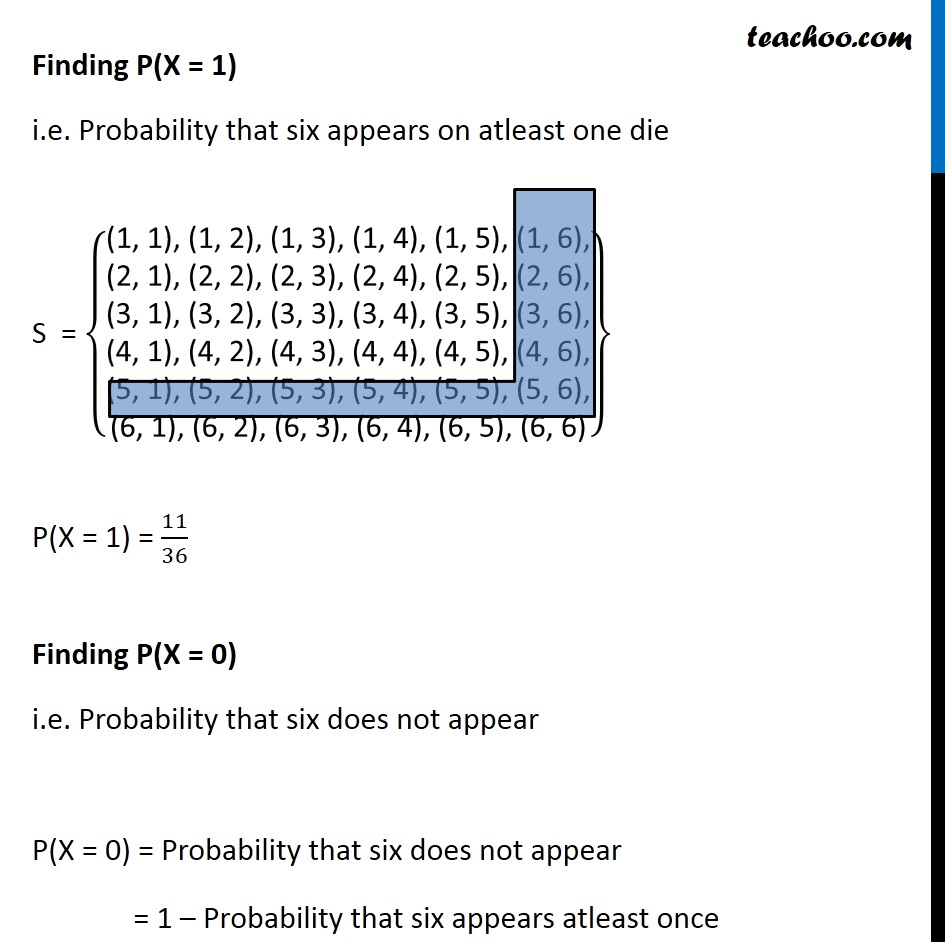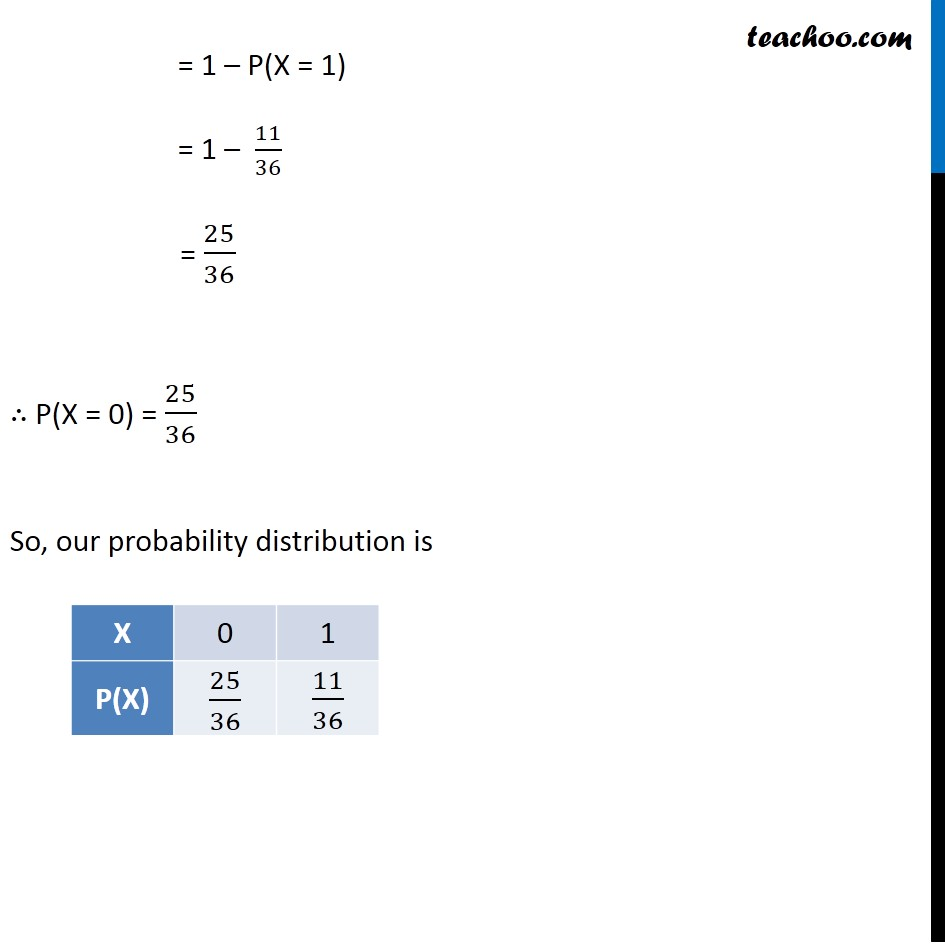Probability Distribution

Chapter 13 Class 12 Probability
Serial order wiseLearn in your speed, with individual attention - Teachoo Maths 1-on-1 Class

### Transcript

Question 5 Find the probability distribution of the number of successes in two tosses of a die, where a success is defined as (ii) six appears on at least one dieSince pair of die are thrown, There can be two cases, Six does not appear at all Six appears on atleast one die Hence, X = 1 means six appears on atleast one die X = 0 means six does not appear Finding P(X = 1) i.e. Probability that six appears on atleast one die S = {█("(1, 1), (1, 2), (1, 3), (1, 4), (1, 5), (1, 6)," @"(2, 1), (2, 2), (2, 3), (2, 4), (2, 5), (2, 6)," @"(3, 1), (3, 2), (3, 3), (3, 4), (3, 5), (3, 6)," @"(4, 1), (4, 2), (4, 3), (4, 4), (4, 5), (4, 6)," @"(5, 1), (5, 2), (5, 3), (5, 4), (5, 5), (5, 6)," @"(6, 1), (6, 2), (6, 3), (6, 4), (6, 5), (6, 6)" )} P(X = 1) = 11/36 Finding P(X = 0) i.e. Probability that six does not appear P(X = 0) = Probability that six does not appear = 1 – Probability that six appears atleast once = 1 – P(X = 1) = 1 – 11/36 = 25/36 ∴ P(X = 0) = 25/36 So, our probability distribution is